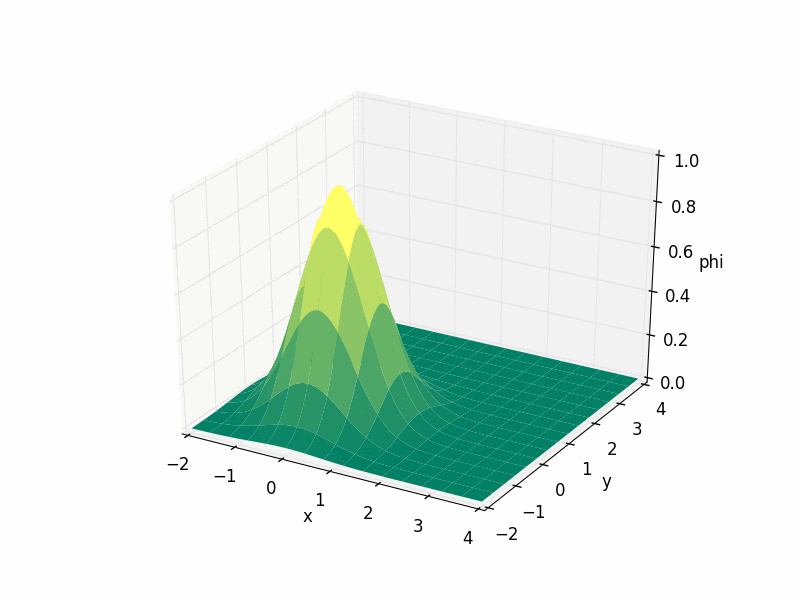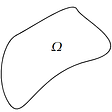# What does the rank of a matrix tell us about the solution?

It was around November 2020 when I gave an interview and was unable to give answers to questions, one of which is the title of this article.

Previously, I have written about another question:

A rank of the matrix is probably the most important concept you learn in Matrix Algebra. There are two ways to look at the rank of a matrix. One from a theoretical setting and the other from an applied setting.

The rank of matrix A is equal to the dimension of the Image of 𝐴 (which is spanned by columns of A).

From a theoretical setting, if we say that a linear operator has a rank p, it means that the range of the linear operator is a 𝑝 dimensional space. From a matrix algebra point of view, column rank denotes the number of independent columns of a matrix while row rank denotes the number of independent rows of a matrix. An interesting, and I think a non-obvious (though the proof is not hard) fact is the row rank is the same as column rank. When we say a matrix 𝐴∈ℝ𝑛×𝑛 has rank p, what it means is that if we take all vectors 𝑥∈ℝ𝑛×1, then Ax spans a p dimensional sub-space.

From an applied setting, the rank of a matrix denotes the information content of the matrix. The lower the rank, the lower is the “information content”. So if we know that a matrix is of low rank, then we can compress and store the matrix and can do efficient matrix operations using it. The above ideas can be extended for any linear operator and these in fact form the basis for various compression techniques. You might also want to look up Singular Value Decomposition which gives us a nice (though expensive) way to make low-rank approximations of a matrix that allows for compression.

From solving a linear system point of view, when the square matrix is rank deficient, it means that we do not have complete information about the system, ergo we cannot solve the system — we can, but not in the usual sense… hence least squares, Tikhonov regularization, and a bunch of other fancy tricks.

A square matrix is said to be of full rank if all of its rows (or columns; these are equivalent) are linearly independent. It can be proved that this is equivalent to saying the matrix is invertible

If a square matrix A is of full rank, there is one solution to the equation Ax=b. If the matrix is not of full rank, then there are infinitely many solutions.

In the latter case, the rank tells you what the dimensionality of the target space of the matrix is — in other words, how much “information” is “preserved” by the matrix, and the nullity how much information is “destroyed”.

# References

--

--

--

## More from Talha Hanif Butt

MS Thesis Student, CVGL, LUMS http://pk.linkedin.com/in/talhahanifbutt

Love podcasts or audiobooks? Learn on the go with our new app.

## TMO Indicator## Approaches To Problem Solving #8 (Binary Search)## The Black Swan effect: why are we so bad at predicting stuff?## Recursively Finding the Nth Fibonacci Number## MATHEMATICS## Talha Hanif Butt

MS Thesis Student, CVGL, LUMS http://pk.linkedin.com/in/talhahanifbutt

## So… what is a simulation? Pt II## Theory’s Death is Highly Exaggerated…## Most efficient way to do Matrix Multiplication —Python vs PyTorch #Deep Learning## Shannon’s Opening Wordle Move